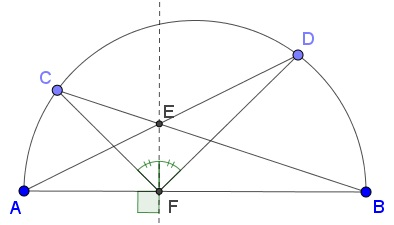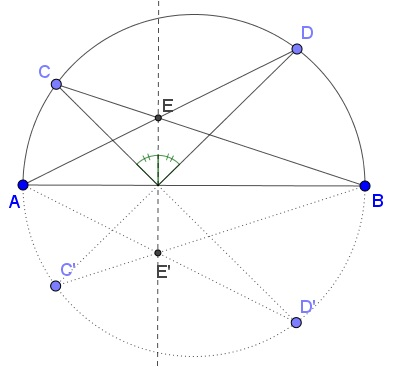# The Mirror Property of Altitudes via Pascal's HexagramWhat is this about? A Mathematical Droodle

ExplanationThe applet may suggest the following statement by Greg Markowsky:

Let points $C$ and $D$ be located on a semicircle with diameter $AB.$ Let $E$ be the intersection of $AD$ and $BC$ and $F$ the foot of the perpendicular from $E$ to $AB.$ Then $EF$ is the bisector of $\angle CFD.$Note that since inscribed angles $ACB$ and $ADB$ subtend a diameter they are right. So that $AD$ and $BC$ are altitudes in $ΔABS$ where $S$ is the intersection of $AC$ and $BD,$ $E$ is the orthocenter of this triangle and $EF$ is the third altitude. What is claimed is nothing more nor less than the mirror property of the altitude. Greg's shows how the latter can be derived from Pascal's theorem of the hexagram.

### Proof

Reflect $C$ and $D$ in $AB$ to obtain $C'$ and $D'.$ Consider the hexagon $ADC'BCD'.$ By Pascal's theorem, the three points at which the pairs $\{AD, BC\},$ $\{DC', CD'\},$ and $\{C'B, D'A\}$ of opposite sides meet, lie on a straight line.The three intersection points are $E,$ $F,$ and $E'.$ This is clear for $E$ and $E'.$ Assuming that $DC'$ and $CD'$ intersect in $F',$ observe that, by symmetry, $EE'$ is orthogonal to $AB$ and, for the same reason, $DC'$ and $CD'$ intersect on $AB,$ the axis of symmetry. Therefore both $F$ and $F'$ lie on $AB$ and also on $EE'$ and, thus, coincide.

### References

1. G. Markowsky, Pascal's Hexagon Theorem implies the Butterfly Theorem, Mathematics Magazine, Volume 84, Number 1, February 2011 , pp. 56-62(7)### Pascal and Brianchon Theorems•